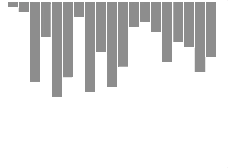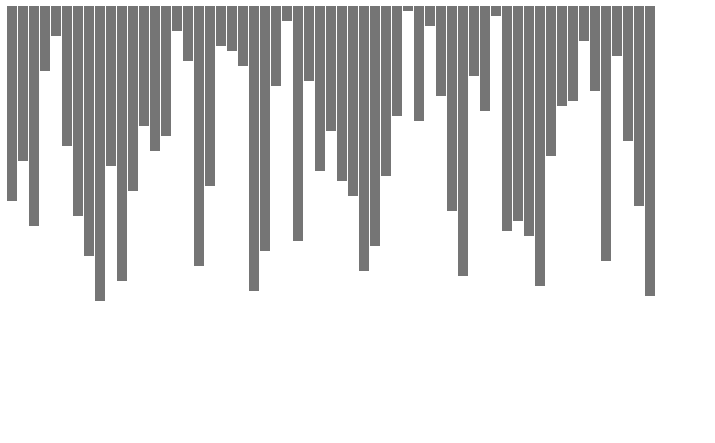For most developers, bubble sort is one of the first algorithms we learn. Therefore, visualizing it can be highly satisfying and feels a bit like meeting an old friend after a long time.

This article will take you through visualizing the bubble sort algorithm using HTML5 canvas API.

If you would like to jump straight to the results and have a look at the code, here is a codepen.

In the meantime, below is a little sneak peek of what we are going to accomplish here.If you want to follow along, run the following command to get the initial boilerplate code generated into your working directory.

 ``````1 `````` ``````npx scaffolder-cli i --from-github https://github.com/galElmalah/scaffolder-canvas-template.git --template canvas && cd visualizing-bubble-sort ``````

This will leverage Scaffolder to create the initial setup.

Or create a basic `index.html` file and `script.js`.

## Now lets jump right ahead and start coding#

All of the javascript code is written in `script.js`.

The first thing we will need is an unsorted array to sort.  Let’s write a helper function for creating shuffled arrays.

Cool. Now, we will write a simple implementation of the bubble sort algorithm.

Next, we’ll get our canvas and create a context.

So we got all the basics covered, and now it’s up to us to decide how to visualize the array.  The most straightforward way is to just draw a rectangle corresponding to each array element, and set the height according to that element value (the higher the value the higher the rectangle will be).

Here is a representation of our custom rectangle.

Let’s test that everything is working as expected, by drawing our shuffled array.

Multiply each height param by 5 to get a nice scale, so each pixel will equal 5 pixels.

We can make the height and width of the rectangle dynamic, by making it span the full height and width of the screen. Try doing this yourself. Here is a working example for the lazy ones (notice the `calcMembersHeightScale` and `calcMembersWidth` functions).

If all goes well, you should see something similar to the following in your browser.Now, let’s go back to our sorting function. What are the actions and states we care about? compare, swap, and sort. Let’s add a custom action dictionary.

Change our bubble sort function to accept an `onAction` callback, and call it when an action is made in our bubble sort with the appropriate action.

## We are almost done so hang in there!#

What should we do in each action? Give the members a different color based on the action, and “move” them if necessary - which will just be swapping their values. Now let’s create an action map, according to our known actions.

We seem to have all of the parts needed in order to visualize this nifty little thing! Let’s give it a try.

I’ll be damned! it seems like we got only the fully sorted state. How can we solve this? we need to time our painting somehow. Let’s add two variables, `speed` which will determine how much time will pass between each step, and `ticks` to count each call to our `onAction` callback.

A couple of things you should notice in the above example:

• Clearing the canvas on each iteration.
• Resetting the color property for all of our rectangles on each iteration.

Now putting it all together, we should end up with something like this.

And there you have it, we just visualized this cool algorithm in 5 minutes!

Hope you enjoyed this little blog post!If you liked this visualization, check out some more sorting algorithms visualizations I created.